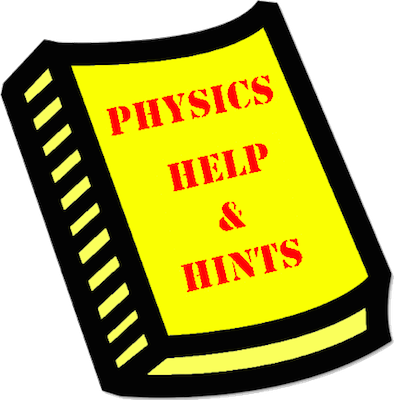# Mission RM7: Concave Mirror Mathematics

Mission RM7 pertains to the use of the mirror equation, the magnification ratio, and algebraic skills to solve Physics word problems. The mission consists of 18 questions organized into 6 Question Groups. You must answer one question from each Question Group to complete the mission. The learning outcomes for this mission are ...

#### Learning Outcomes

• The student should be able to use the mirror equation to predict the image distance if the object distance and focal length are known (or to predict the focal length which is required to produce a known image and object distance). (For concave mirrors).
• The student should be able to combine the mirror equation and magnification ratio to predict either the image distance or image height and magnification for a known object distance and focal length. (For concave mirrors).

### Launch Mission RM7

#### Getting Help

If you are not familiar with this topic, then you should first learn about the topic using our written Tutorial or our Video Tutorial:

The Physics Classroom, Reflection and Mirrors Unit, Lesson 3, Part f

#### Video Tutorial:

The Mirror Equation and Magnification Ratio

####Question-Specific Help

Each Question Group has its own Help page with information specific to the question. You can access the Help page from within the mission by tapping on the Help Me! icon (textbook). For your convenience, links to those pages are provided below: